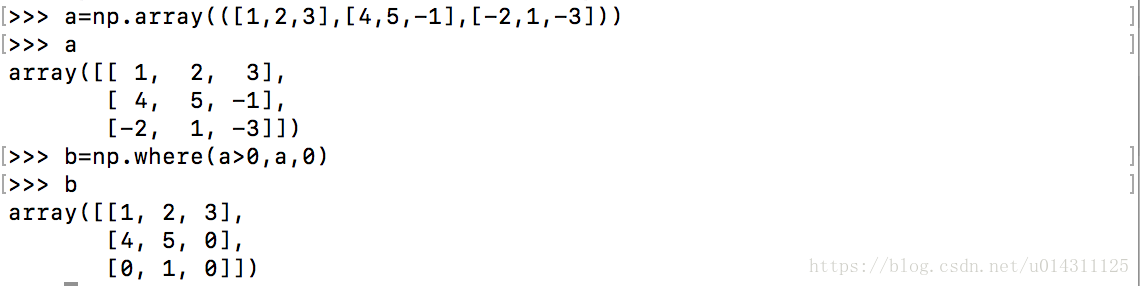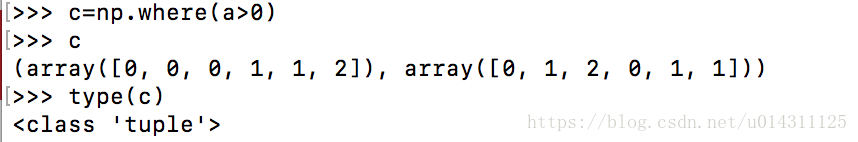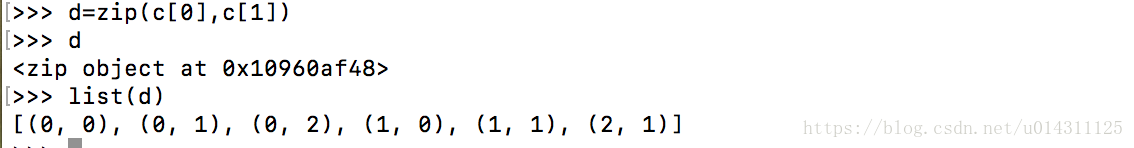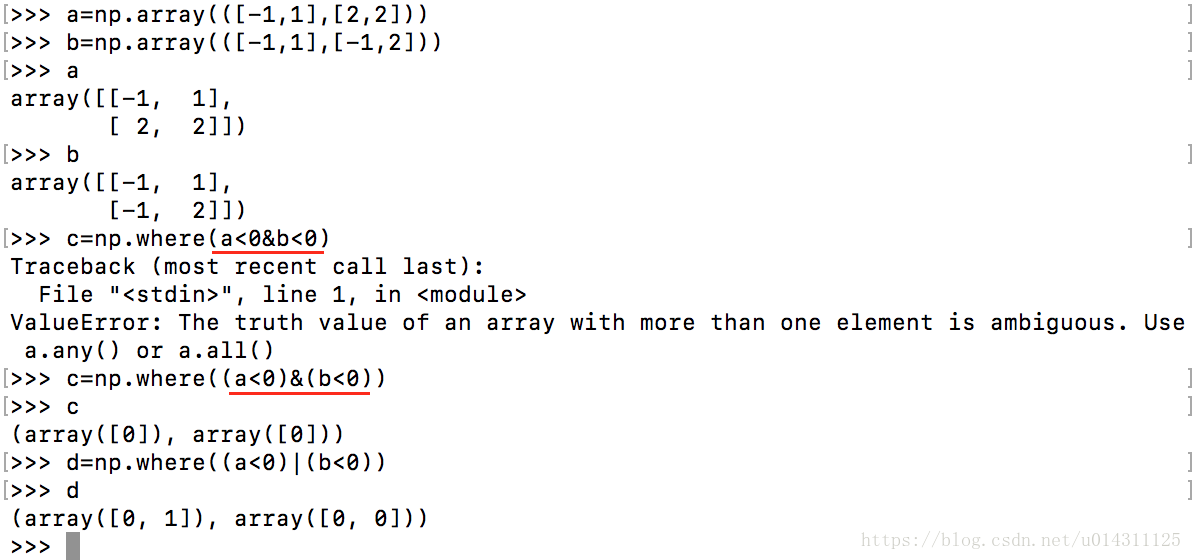## numpy.where() 用法详解

2633人浏览 / 0人评论

numpy.where (condition[, x, y])

numpy.where() 有两种用法：

1. np.where(condition, x, y)

```>>> aa = np.arange(10)
>>> np.where(aa,1,-1)
array([-1, 1, 1, 1, 1, 1, 1, 1, 1, 1]) # 0为False，所以第一个输出-1
>>> np.where(aa > 5,1,-1)
array([-1, -1, -1, -1, -1, -1, 1, 1, 1, 1])

>>> np.where([[True,False], [True,True]],  # 官网上的例子
[[1,2], [3,4]],
[[9,8], [7,6]])
array([[1, 8],
[3, 4]])

```

```>>> a = 10
>>> np.where([[a > 5,a < 5], [a == 10,a == 7]],
[["chosen","not chosen"], ["chosen","not chosen"]],
[["not chosen","chosen"], ["not chosen","chosen"]])

array([['chosen', 'chosen'],
['chosen', 'chosen']], dtype='
```

2. np.where(condition)

```>>> a = np.array([2,4,6,8,10])
>>> np.where(a > 5)       # 返回索引
(array([2, 3, 4]),)
>>> a[np.where(a > 5)]       # 等价于 a[a>5]
array([ 6, 8, 10])

>>> np.where([[0, 1], [1, 0]])
(array([0, 1]), array([1, 0]))

```

```>>> a = np.arange(27).reshape(3,3,3)
>>> a
array([[[ 0, 1, 2],
[ 3, 4, 5],
[ 6, 7, 8]],

[[ 9, 10, 11],
[12, 13, 14],
[15, 16, 17]],

[[18, 19, 20],
[21, 22, 23],
[24, 25, 26]]])

>>> np.where(a > 5)
(array([0, 0, 0, 1, 1, 1, 1, 1, 1, 1, 1, 1, 2, 2, 2, 2, 2, 2, 2, 2, 2]),
array([2, 2, 2, 0, 0, 0, 1, 1, 1, 2, 2, 2, 0, 0, 0, 1, 1, 1, 2, 2, 2]),
array([0, 1, 2, 0, 1, 2, 0, 1, 2, 0, 1, 2, 0, 1, 2, 0, 1, 2, 0, 1, 2]))

# 符合条件的元素为
[ 6, 7, 8]],

[[ 9, 10, 11],
[12, 13, 14],
[15, 16, 17]],

[[18, 19, 20],
[21, 22, 23],
[24, 25, 26]]]

```

1、numpy.where的返回结果

numpy.where调用方式为numpy.where(condition,1,2)

例如通过where()函数将a数组中负值设为0，正值不变2、numpy.where多条件查询#### 全部评论2017-10-01 18:00wjmyly7336064
2017-10-01 18:00

2017-10-01 18:00